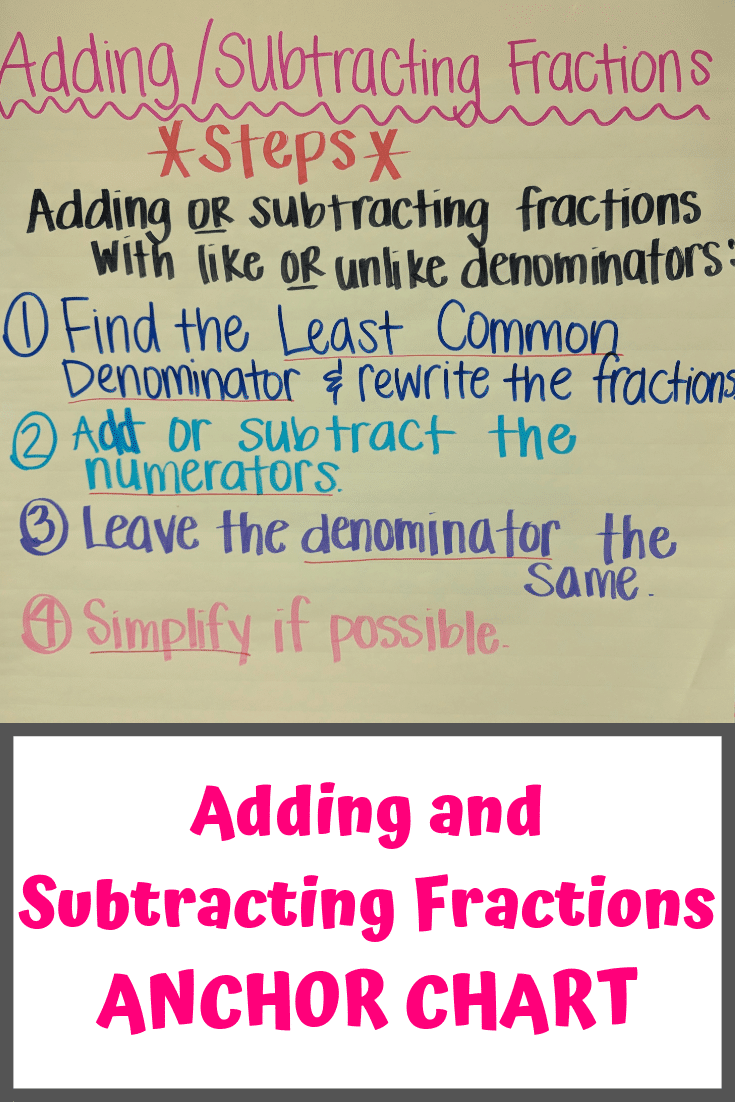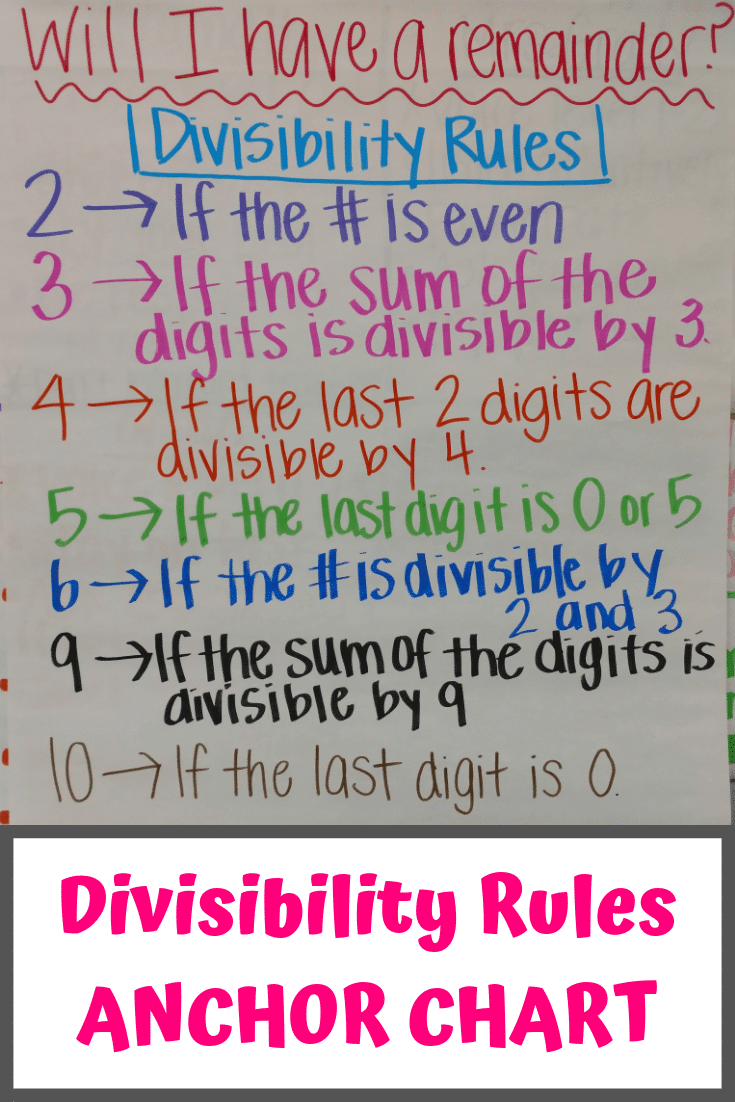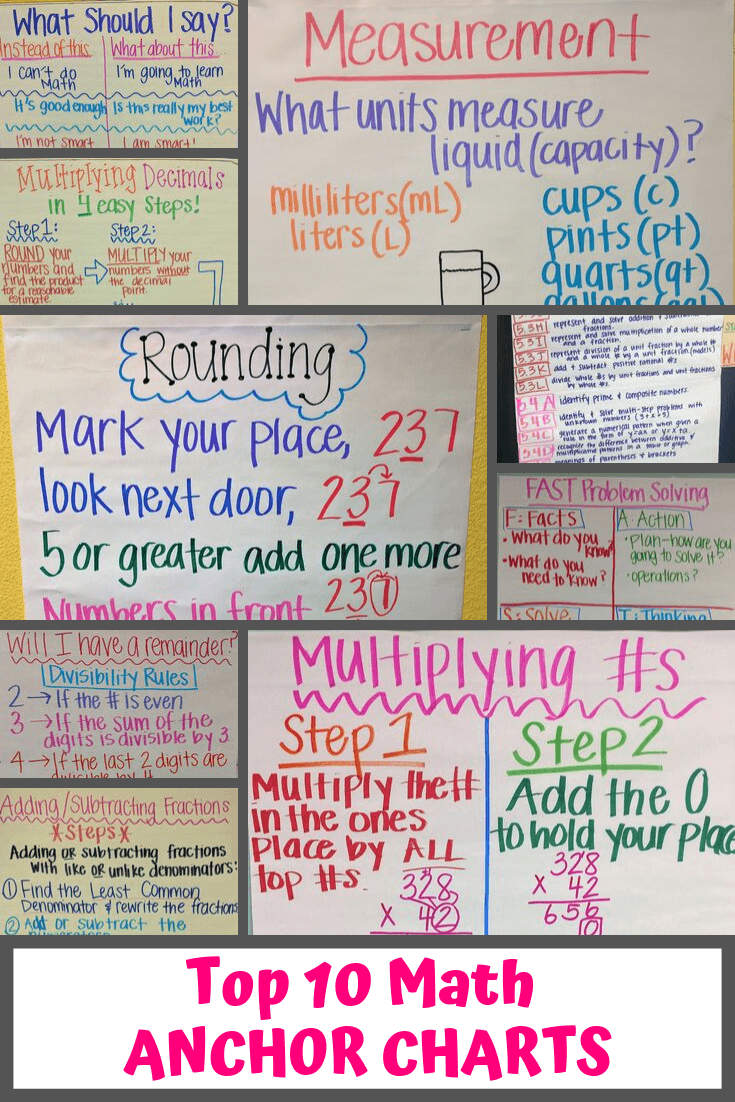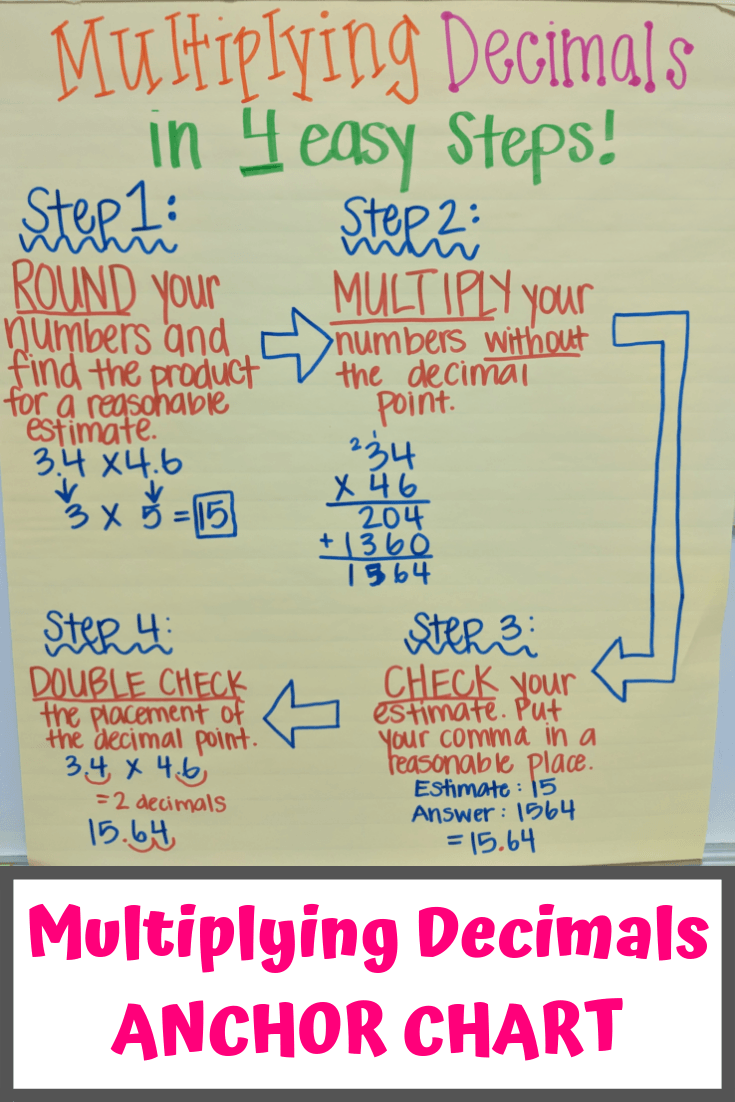# Teaching

Best teaching tips, tricks, and anchor charts.  Especially for elementary teachers, especially math teachers.## Adding Fractions Anchor Chart for Elementary Math Classroom

One visual teaching tool I like to have in my elementary school math classroom is this anchor chart for fractions. It helps students master the process of adding and subtracting fractions, which can be a difficult concept for some students.  Mastering this building block concept is important, because teaching more complicated math involving fractions rests…## Divisibility Rules Anchor Chart for Fifth Grade Math

When teaching division to my fifth graders, I have found that having a divisibility rules anchor chart in my classroom is very helpful!  Division and decimals seem to be two concepts that are difficult for my fifth-grade math students to understand. When I can get my students to remember these divisibility rules, they do significantly…## Rounding Decimals Anchor Chart for Fifth Grade Math Classroom

I used to teach third-grade math, but now I’ve moved to fifth-grade math. Whereas in third grade we were working on rounding whole numbers, in fifth grade we are working on rounding decimals to the nearest whole number, tenth, or hundredth. To help my students understand this concept better, I made this rounding decimals anchor…## 10 Best Math Anchor Charts for Elementary School Classrooms

Math anchor charts are one of the most powerful teaching strategies in my classroom.  My goal with an anchor chart is to help students understand, visualize, and memorize how to solve math problems and understand math concepts. I wholeheartedly believe that anchor charts work.  Time and time again during tests, I see students looking towards…## Multiplying Decimals Anchor Chart for Elementary Math Classroom

Teaching elementary math students to multiply decimals can be difficult for us teachers!  So often, students see a decimal point and they forget that multiplying decimals isn’t a foreign concept if they just take a moment to think about the process.  And there is no reason to just place the decimal randomly! To help my…